# Rational Maps

This formula makes Julia sets from the following class of functions:

`zk+1 = zkP + c - λzk-Q`

P is >= 2 while Q is <= -1. The + c is optional and allows us to perturb familiar Julia sets in interesting ways. Simply varying λ, P and Q allows us to produce a bewildering variety of forms. Credit is due to Robert Devaney for introducing me to the formula.

Despite the superficial resemblance to the standard Julia set formulas for zP (and if λ is 0, it is the same formula), the results are very different. If you load up the Rational map formula with all defaults, this is what you'll see: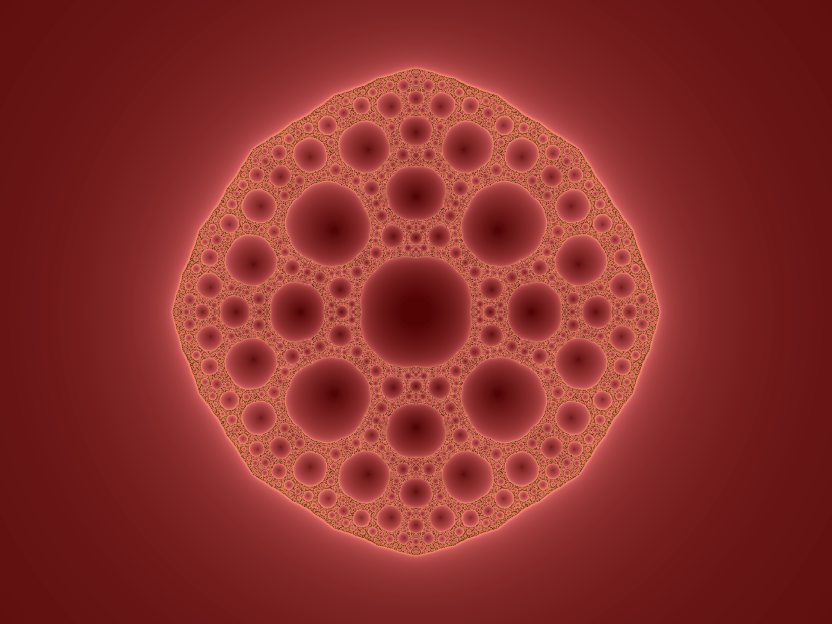Julia set of z2 - 0.0625z-2

The most obvious feature is that it's full of holes! The fractal is homemorphic to (topologically the same as) the Sierpinski carpet:Sierpinski carpet (credit: Wikimedia Commons)

With Q = -1, it is possible to produce a homeomorph of the Sierpinski gasket: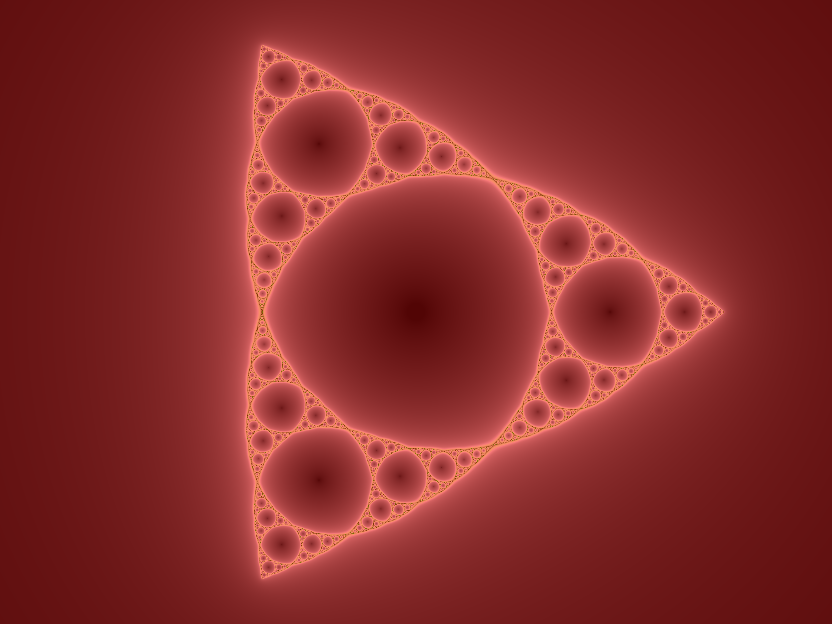Julia set of z2 - 0.59255z-1

So long as c = 0, the order of symmetry is P + |Q|. This combination of parameters shows perfect four-fold symmetry: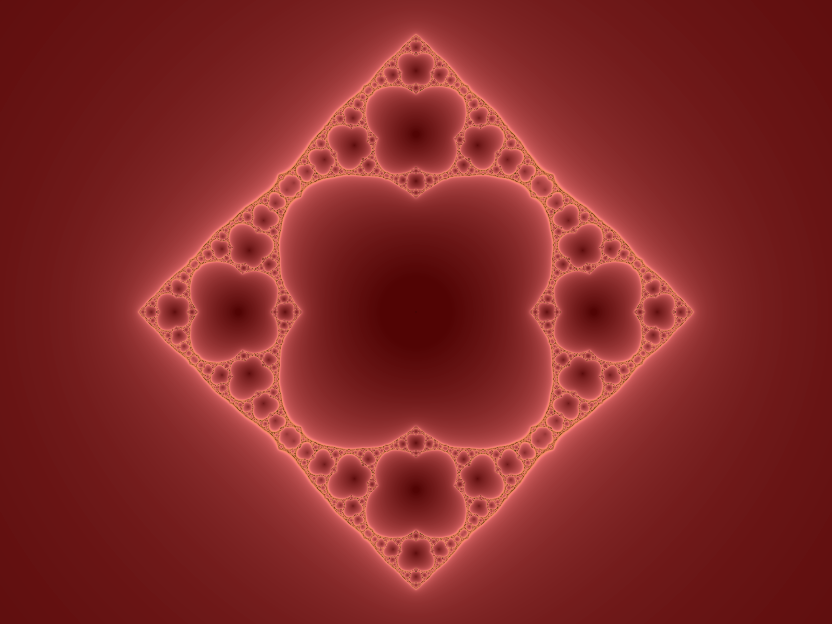Julia set of z2 - 0.35z-2

## Topology of the Rational Map Fractals

The zP + c Julia sets have essentially two forms: a Cantor set (an infinity of scattered points) or a connected set (topologically equivalent to a closed circle). If 0 escapes to ∞ under iteration, J(f) is a Cantor set. When P and |Q| are both > 2, the sets formed by the rational map formula can take three forms:

When z is large, the reciprocal term becomes insignificant and all iterations tend to ∞. The basin of attraction of infinity is denoted by Bλ. Because of the reciprocal term, 0 maps to ∞ after a single iteration. If the basin of attraction around 0 is enclosed by J(f) we denote it by Tλ since it is the trapdoor through which points inside J(f) escape. In addition, there are P + |Q| additional critical points which are solutions of fʹ(λ) = 0. Because of the symmetry of the function, we only need to consider one of these which we denote by νλ.

### Cantor Set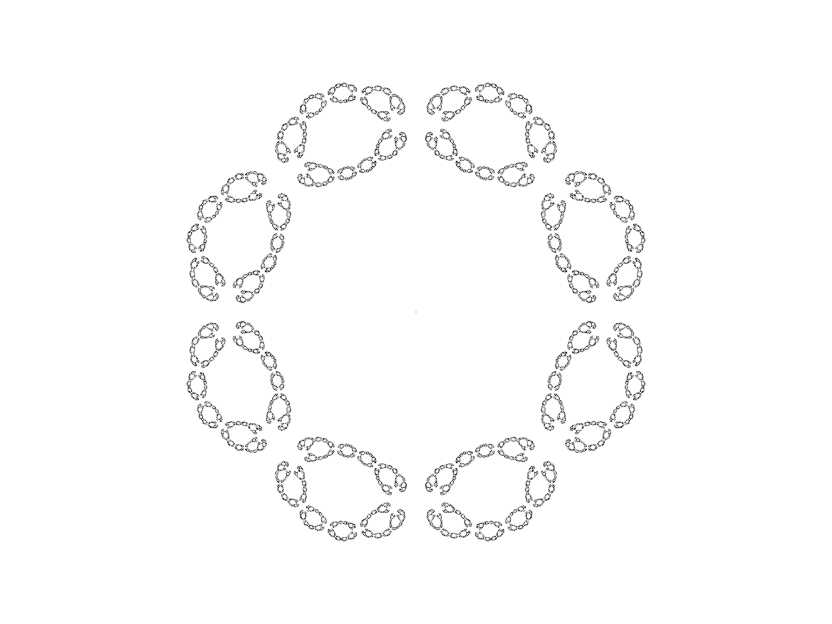Julia set of z4 + 0.2z-4

Here νλ lies inside Bλ.

### Cantor Circles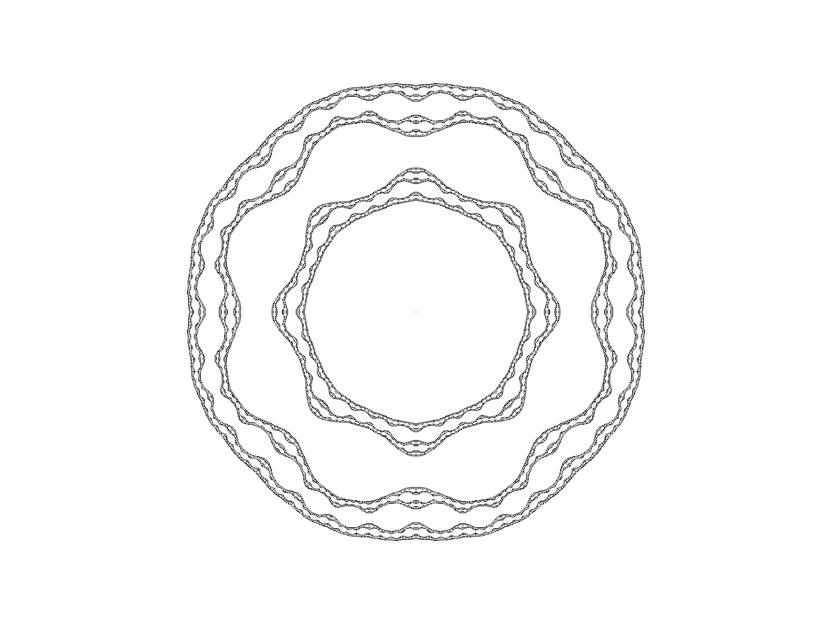Julia set of z4 + 0.05z-4

When νλ lies inside Tλ, J(f) takes the form of concentric, disjoint, simple closed curves.

### Sierpinski Curve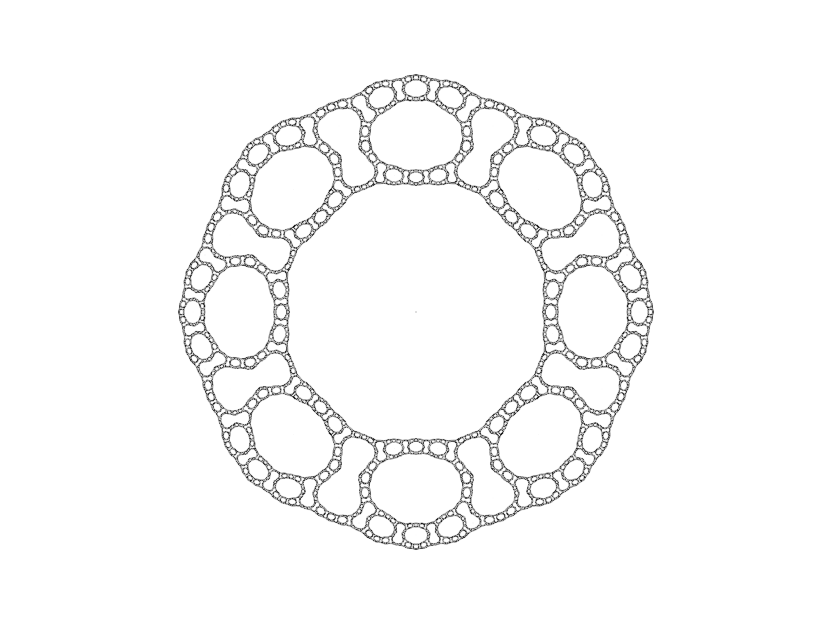Julia set of z4 - 0.1z-4

Otherwise J(f) is connected. If νλ ends up in Tλ under iteration, the form taken is a Sierpinski curve.

## Colouring the Sets

Because the structures are delicate, the default settings de-emphasize the boundary. However, when points in J(f) are closely packed, showing only the boundary greatly improves the appearance, for example: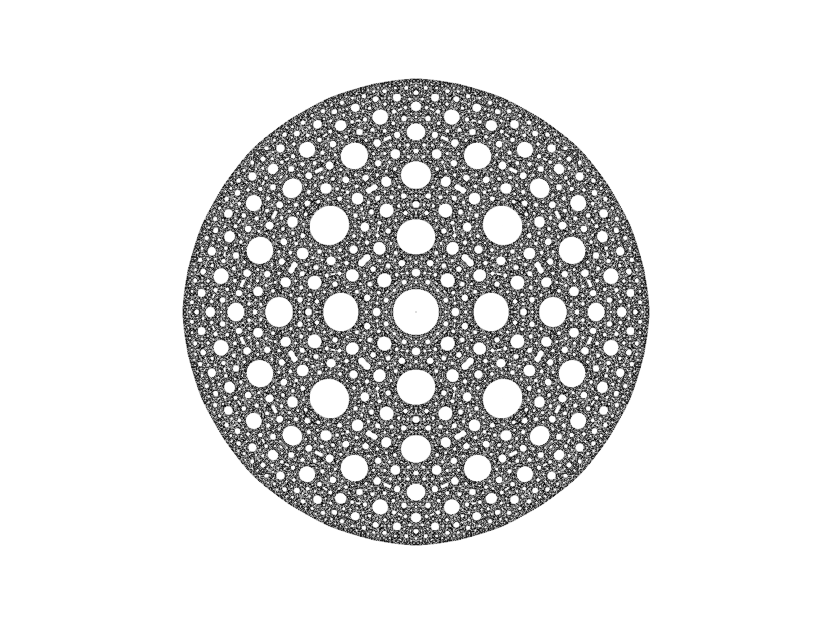Julia set of z2 - 0.01z-2

Exterior colouring for this one looks distinctly messy.

## + c: Perturbing Julia Sets

With c ≠ 0, we are able to put an entirely new spin on familiar forms: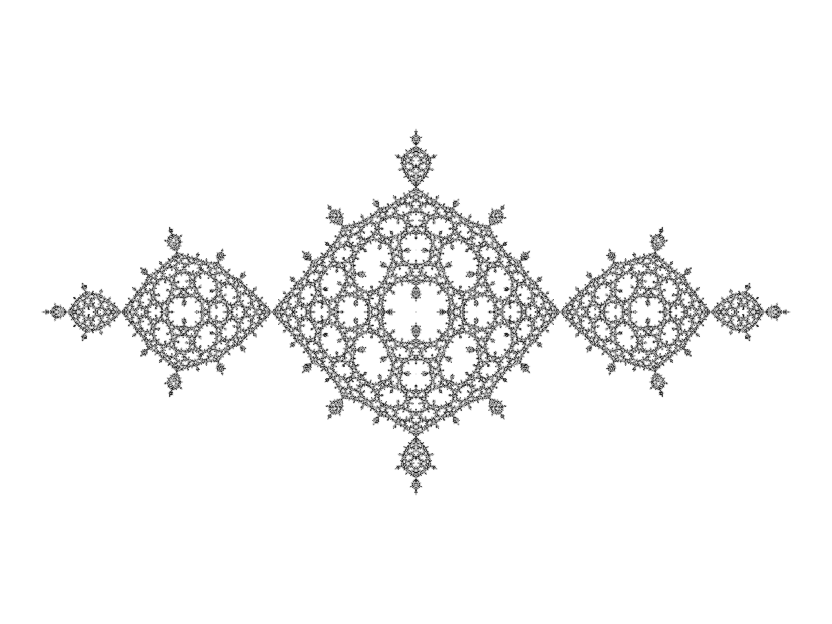Julia set of z2 - 1 - 0.005z-2

## Exploring Further

Unlike, say, the Phoenix formula which is hard to get anything out of, finding cool forms with this formula is as easy as falling off a log. Pretty much any combination of parameters will get you in the ballpark of something interesting and/or attractive. You've got four parameters to play with. Go wild!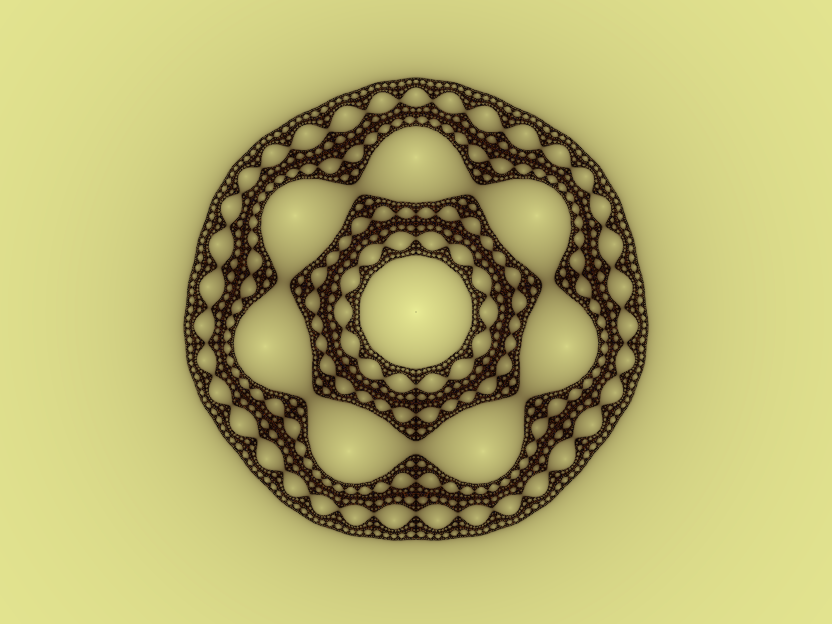Julia set of z5 - 0.06iz-2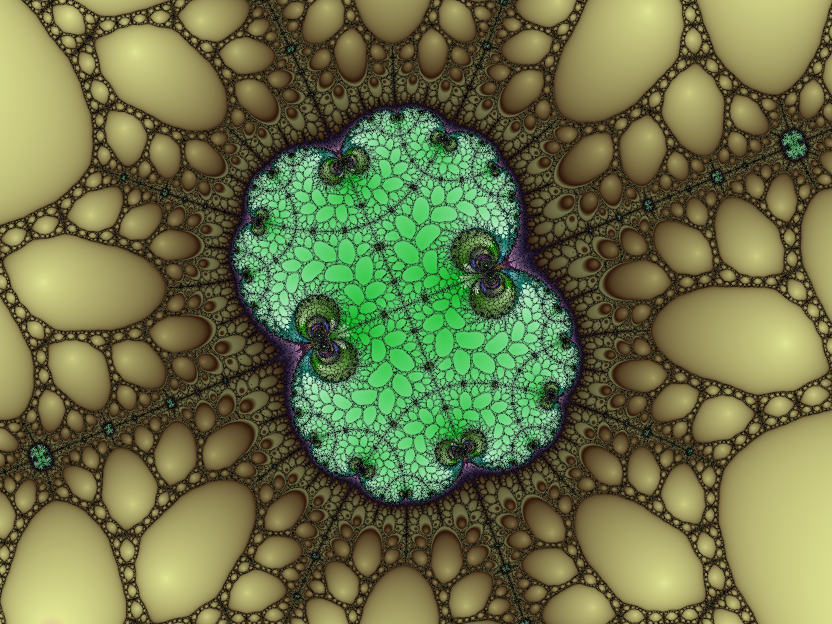Julia set of z3 - 0.0352z-5 (60x zoom)
Back to the index